# Introduction to PID

The PID controller is a commonly used feedback controller consisting of proportional, integral, and derivative terms, hence the name. This article will build up the definition of a PID controller term by term while trying to provide some intuition for how each of them behaves.

First, we’ll get some nomenclature for PID controllers out of the way. The is called the setpoint (the desired position) and the is called the (the measured position). Below are some common variable naming conventions for relevant quantities.

 $$r(t)$$ setpoint $$u(t)$$ control input $$e(t)$$ error $$y(t)$$ output

The $$e(t)$$ is $$r(t) - y(t)$$.

For those already familiar with PID control, this book’s interpretation won’t be consistent with the classical intuition of «past», «present», and «future» error. We will be approaching it from the viewpoint of modern control theory with proportional controllers applied to different physical quantities we care about. This will provide a more complete explanation of the derivative term’s behavior for constant and moving .

The proportional term drives the position error to zero, the derivative term drives the velocity error to zero, and the integral term accumulates the area between the and plots over time (the integral of position ) and adds the current total to the . We’ll go into more detail on each of these.

## Proportional Term

The Proportional term drives the position error to zero.

$u(t) = K_p e(t)$

where $$K_p$$ is the proportional gain and $$e(t)$$ is the error at the current time $$t$$.

The below figure shows a block diagram for a controlled by a P controller.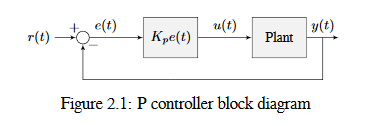Proportional gains act like a «software-defined springs» that pull the toward the desired position. Recall from physics that we model springs as $$F = - kx$$ where $$F$$ is the force applied, $$k$$ is a proportional constant, and $$x$$ is the displacement from the equilibrium point. This can be written another way as $$F = k(0-x)$$ where $$0$$ is the equilibrium point. If we let the equilibrium point be our feedback controller’s , the equations have a one to one correspondence.

$\begin{split}F &= k(r - x) \\ u(t) &= K_p e(t) = K_p(r(t) - y(t))\end{split}$

so the «force» with which the proportional controller pulls the toward the is proportional to the , just like a spring.

## Derivative Term

The Derivative term drives the velocity error to zero.

$u(t) = K_p e(t) + K_d \frac{de}{dt}$

where $$K_p$$ is the proportional gain, $$K_d$$ is the derivative gain, and $$e(t)$$ is the error at the current time $$t$$.

The below figure shows a block diagram for a controlled by a PD controller.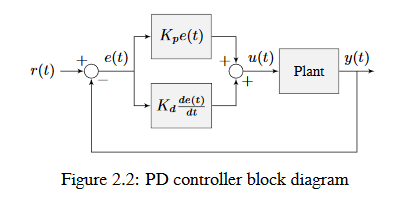A PD controller has a proportional controller for position ($$K_p$$) and a proportional controller for velocity ($$K_d$$). The velocity is implicitly provided by how the position changes over time. To prove this, we will rearrange the equation for a PD controller.

$u_k = K_p e_k + K_d \frac{e_k - e_{k-1}}{dt}$

where $$u_k$$ is the at timestep $$k$$ and $$e_k$$ is the at timestep $$k$$. $$e_k$$ is defined as $$e_k = r_k - x_k$$ where $$r_k$$ is the and $$x_k$$ is the current at timestep $$k$$.

$\begin{split}u_k &= K_p (r_k - x_k) + K_d \frac{(r_k - x_k) - (r_{k-1} - x_{k-1})}{dt} \\ u_k &= K_p (r_k - x_k) + K_d \frac{r_k - x_k - r_{k-1} + x_{k-1}}{dt} \\ u_k &= K_p (r_k - x_k) + K_d \frac{r_k - r_{k-1} - x_k + x_{k-1}}{dt} \\ u_k &= K_p (r_k - x_k) + K_d \frac{(r_k - r_{k-1}) - (x_k - x_{k-1})}{dt} \\ u_k &= K_p (r_k - x_k) + K_d \left(\frac{r_k - r_{k-1}}{dt} - \frac{x_k - x_{k-1}}{dt}\right)\end{split}$

Notice how $$\frac{r_k - r_{k-1}}{dt}$$ is the velocity of the . By the same reason, $$\frac{x_k - x_{k-1}}{dt}$$ is the velocity at a given timestep. That means the $$K_d$$ term of the PD controller is driving the estimated velocity to the velocity.

If the is constant, the implicit velocity is zero, so the $$K_d$$ term slows the down if it’s moving. This acts like a «software-defined damper». These are commonly seen on door closers, and their damping force increases linearly with velocity.

## Integral Term

Importante

Integral gain is generally not recommended for FRC® use. There are better approaches to fix like using feedforwards or constraining when the integral control acts using other knowledge of the .

The Integral term accumulates the area between the and plots over time (i.e., the integral of position ) and adds the current total to the . Accumulating the area between two curves is called integration.

$u(t) = K_p e(t) + K_i \int_0^t e(\tau) \,d\tau$

where $$K_p$$ is the proportional gain, $$K_i$$ is the integral gain, $$e(t)$$ is the error at the current time $$t$$, and $$\tau$$ is the integration variable.

The Integral integrates from time $$0$$ to the current time $$t$$. we use $$\tau$$ for the integration because we need a variable to take on multiple values throughout the integral, but we can’t use $$t$$ because we already defined that as the current time.

The below figure shows a block diagram for a controlled by a PI controller.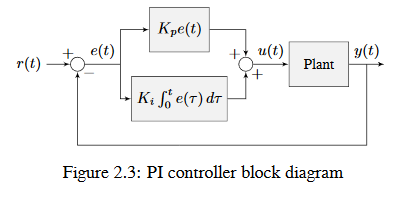When the is close the in steady-state, the proportional term may be too small to pull the all the way to the , and the derivative term is zero. This can result in as shown in figure 2.4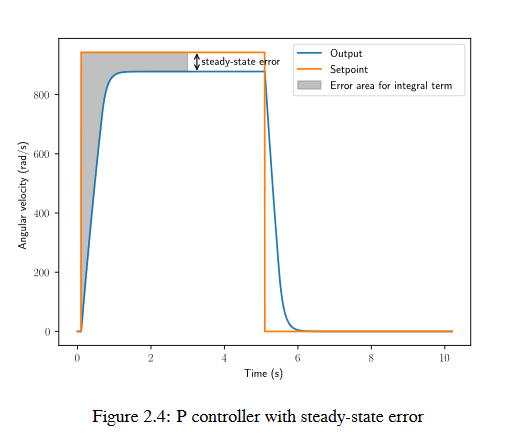A common way of eliminating is to integrate the and add it to the . This increases the until the converges. Figure 2.4 shows an example of for a flywheel, and figure 2.5 shows how an integrator added to the flywheel controller eliminates it. However, too high of an integral gain can lead to overshoot, as shown in figure 2.6.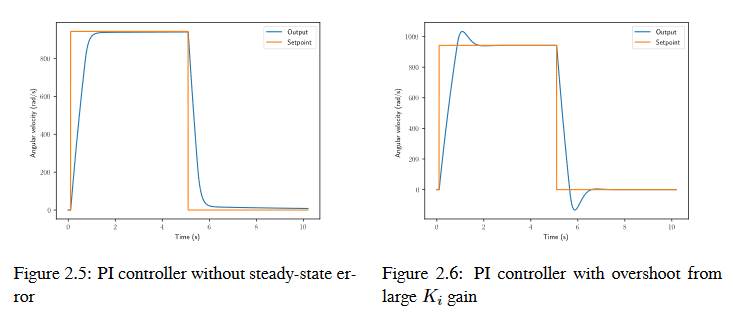## PID Controller Definition

Nota

For information on using the WPILib provided PIDController, see the relevant article.

When these terms are combined, one gets the typical definition for a PID controller.

$u(t) = K_p e(t) + K_i \int_0^t e(\tau) \,d\tau + K_d \frac{de}{dt}$

where $$K_p$$ is the proportional gain, $$K_i$$ is the integral gain, $$K_d$$ is the derivative gain, $$e(t)$$ is the error at the current time $$t$$, and $$\tau$$ is the integration variable.

The below figure shows a block diagram for a PID controller.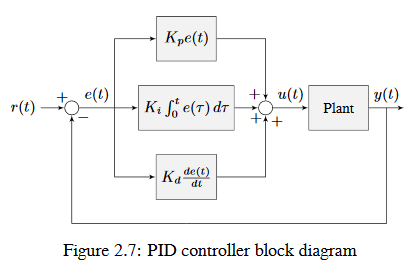## Response Types

A driven by a PID controller generally has three types of responses: underdamped, over-damped, and critically damped. These are shown in figure 2.8.

For the in figure 2.7, is the time the takes to initially reach the reference after applying the . is the time the takes to settle at the after the is applied.

An underdamped response oscillates around the before settling. An overdamped response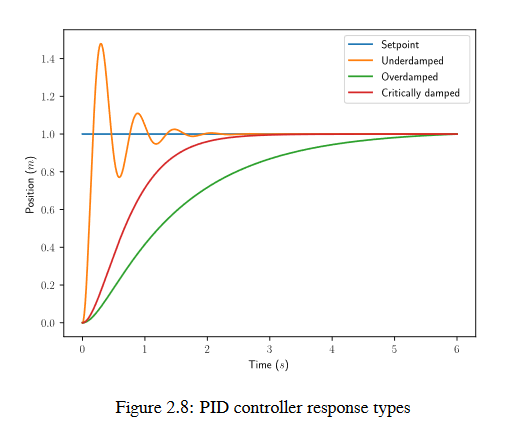is slow to rise and does not overshoot the . A critically damped response has the fastest without overshooting the .